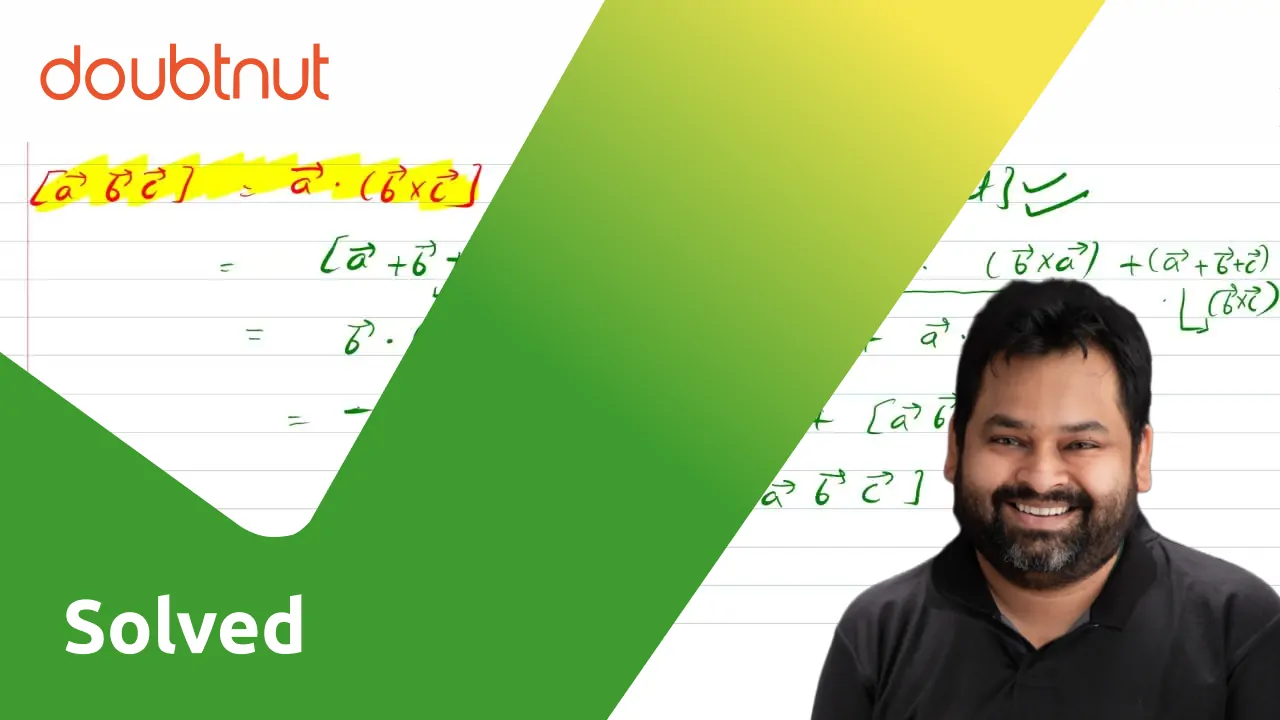Doubtnut App - Get 50 Lakh+ video solutions better than GoogleInstall Now

# If →a,→band→c are three non-coplanar vectors, then (→a+→b+→c).[(→a+→b)×(→a+→c)] equals

A

0

B

[abc]

C

2[abc]

D

[abc]

Text Solution
Verified by Experts

## (→a+→b+→c).[(→a+→b)×(→a+→c)] =(→a+→b+→c).[→a×→a+→a×→c+→b×→a+→b×→c] =(→a+→b+→c).[→a×→c+→b×→a+→b×→c] [→a→b→c]−[→a→b→c]−[→a→b→c] −[→a→b→c]